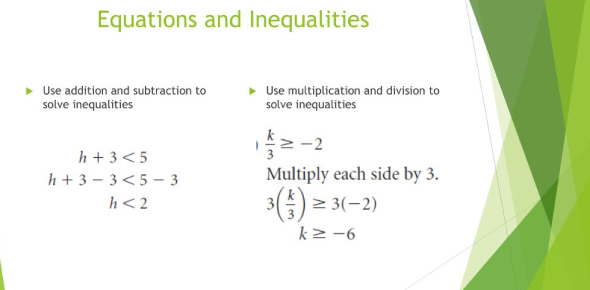# Equations And Inequalities Quiz Questions And Answers

15 Questions | Total Attempts: 2084SettingsCreate your own QuizHave you practiced inequalities well during mathematics class? Take this quiz on equations and inequalities with questions and answers to test your knowledge. We have got some basic questions on equations as well as inequalities for your practice. Start the quiz, and see how well you perform with the final scores. All the best! Try to get a perfect score on this quiz. You can share the quiz with others and challenge them to see who scores better on this algebra quiz.

• 1.
Solve for y:y - 5 = 12
• A.

Y = 7

• B.

Y = -7

• C.

Y = 17

• D.

Y = -17

• 2.
Solve for g:2g + 1 = 13
• A.

G = 6

• B.

G = 7

• C.

G = 9

• D.

G = 5

• 3.
Solve for k:-3k - 2 = 10
• A.

K = 4

• B.

K = -3

• C.

K = -4

• D.

K = 3

• 4.
Check all of the answers that are showing inequalities:
• A.

X = 1

• B.

X < 5

• C.

7 > k

• D.

-2 ≥ u

• E.

8 = w

• F.

F ≤ -2

• G.

H = -1

• 5.
An inequality means that the variable can only be one number.  For example, x can only be 6.
• A.

True

• B.

False

• 6.
Solve this inequality:7y < 14
• A.

Y < -2

• B.

Y < 2

• C.

Y > 2

• D.

Y > -2

• 7.
Solve this inequality:g - 5 ≤ 2
• A.

G ≤ -3

• B.

G < -3

• C.

G < 7

• D.

G ≤ 7

• 8.
Solve for d:6d - 1 > 17
• A.

D > -3

• B.

D < 3

• C.

D > 3

• D.

D < -3

• 9.
Solve for x:-2x + 6 ≤ 20
• A.

X ≥ -7

• B.

X ≥ 7

• C.

X ≤ -7

• D.

X ≤ 7

• 10.
Check all of the "clue words" that mean multiply:
• A.

Times

• B.

Split equally

• C.

Product

• D.

Less than

• 11.
Write an algebraic expression for: The product of 6 and y
• 12.
Evaluate for x = 2 and y = -1:3x + 2y + x
• A.

= 0

• B.

= 10

• C.

= 6

• 13.
Which inequality is represented by this graph:
• A.

X ≤ -2

• B.

X < -2

• C.

X ≥ -2

• D.

X > -2

• 14.
Solve for x: -6x = 36
• A.

X = 6

• B.

X = -6

• C.

X = 30

• D.

X = 42

• 15.
Which inequality is represented by this graph:
• A.

X < 3

• B.

X > 3

• C.

X ≥ 3

• D.

X ≤ 3

Related TopicsBack to top
×

Wait!
Here's an interesting quiz for you.